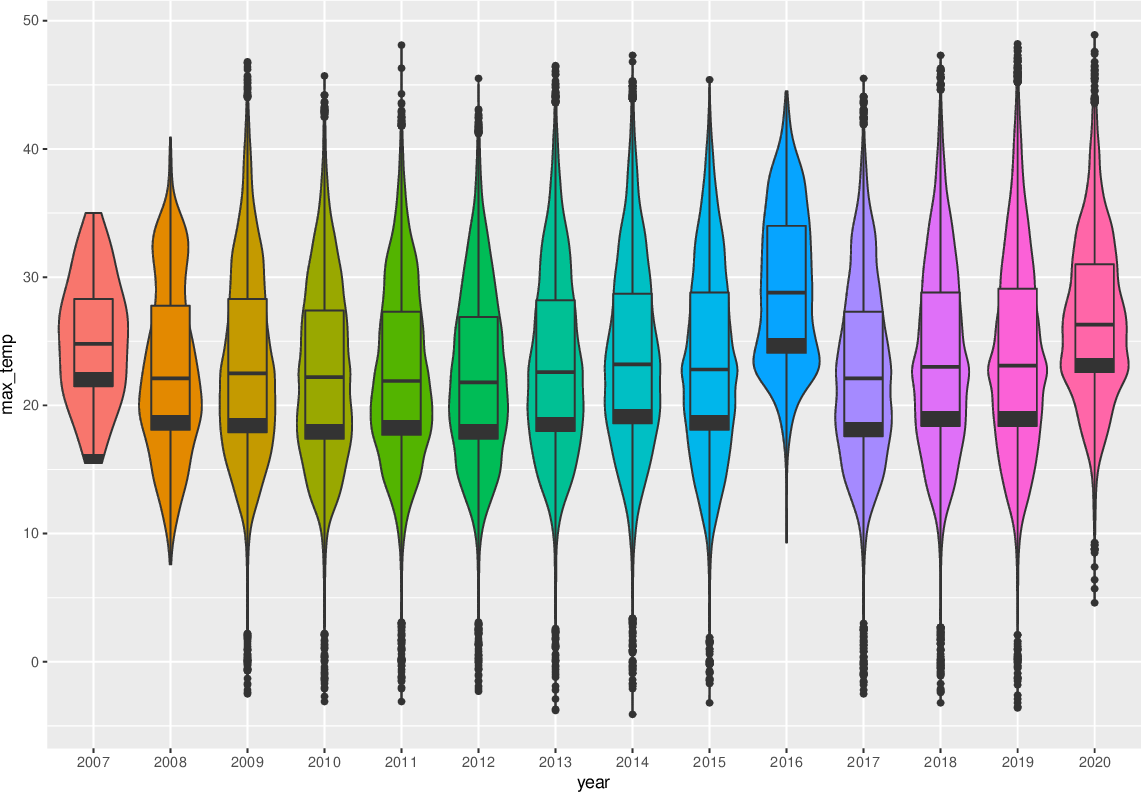Data Science Desktop Survival Guide by Graham WilliamsDesktop Survival Project Home Preface Data Science Introducing R R Constructs R Tasks R Strings R Read, Write, and Create Data Template Data Exploration Data Wrangling Data Visualisation Statistics ML Template ML Scenarios ML Activities ML Applications ML Algorithms Cluster Analysis Decision Trees Computer Vision Graph Data Privacy Literate Data Science Coding with Style Resources Bibliography Index

## Violin Plot Embedded Box Plot

Rawds                                                                    %>%   mutate(year=factor(format(ds\$date, "%Y")))                          %>%   ggplot(aes(x=year, y=max_temp, fill=year))                           +   geom_violin()                                                        +   geom_boxplot(width=.5, position=position_dodge(width=0))             +   theme(legend.position="none") We can overlay the violin plot with a box plot to show the quartiles.